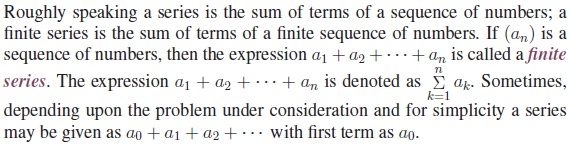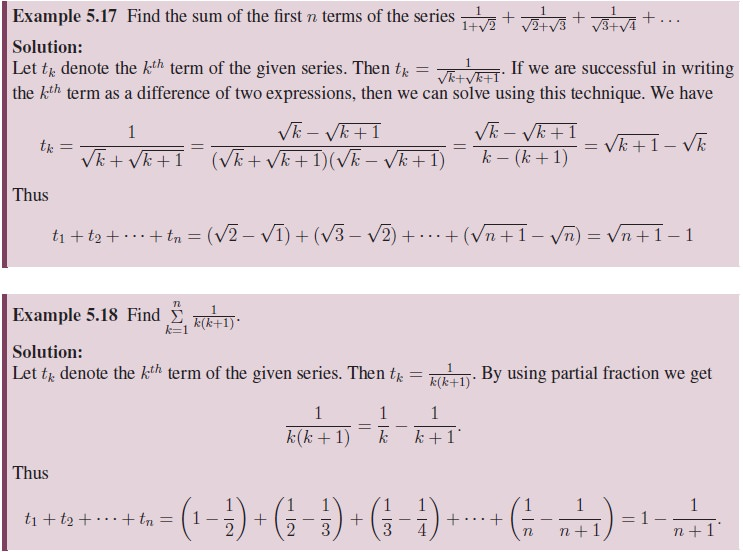Home | | Maths 11th std | Finite Series

# Finite Series

In the earlier classes we studied about the sum of a few terms, like sum of first n terms, of arithmetic and geometric progressions.

Finite Series## 1. Sum of Arithmetic, Geometric and Arithmetico-Geometric Progressions

In the earlier classes we studied about the sum of a few terms, like sum of first n terms, of arithmetic and geometric progressions. We now recall them.

### Sum of Arithmetic and Geometric Progressions### Sum of Arithmetico-Geometric Progressions## 2. Telescopic Summation for Finite Series

Telescopic summation is a more general method used for summing a series either for finite or infinite terms. This technique expresses sum of n terms of a given series just in two terms, usually first and last term, by making the intermediate terms cancel each other. After canceling intermediate terms, we bring the last term which is far away from the first term very close to the first term. So this process is called “Telescopic Summation”.## 3. Some Special Finite Series

In this section we give some of the important formulas of summing up finitely many terms which follows either an AP, GP, or any specific series.Note that the above three results were proved in the earlier classes.

Tags : Definition, Formula, Solved Example Problems, Exercise | Mathematics , 11th Mathematics : UNIT 5 : Binomial Theorem, Sequences and Series
Study Material, Lecturing Notes, Assignment, Reference, Wiki description explanation, brief detail
11th Mathematics : UNIT 5 : Binomial Theorem, Sequences and Series : Finite Series | Definition, Formula, Solved Example Problems, Exercise | Mathematics